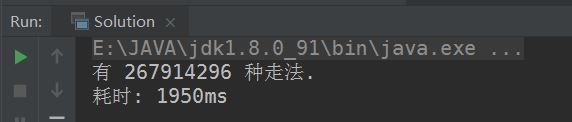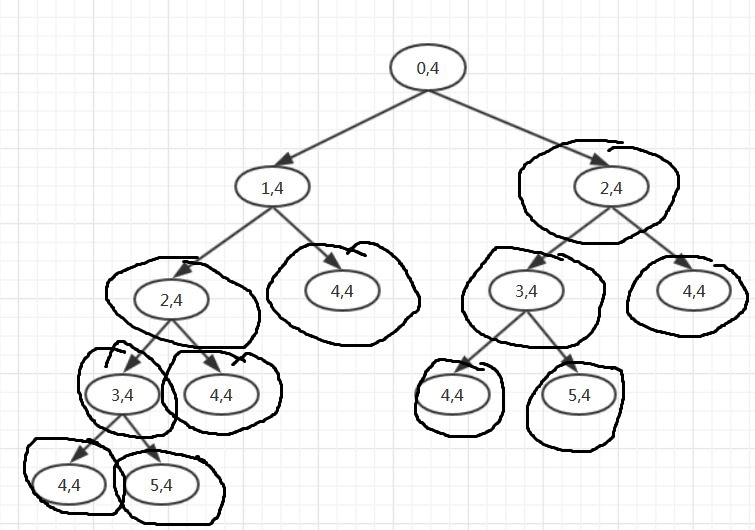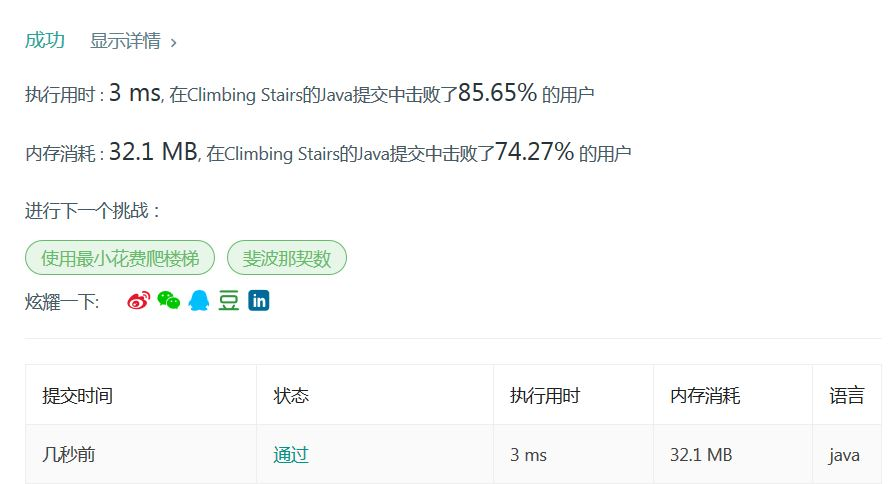## 遇到一道有意思的算法题 --- 爬楼梯

April . 11 . 2019
• 起因

``````输入： 2

1.  1 阶 + 1 阶
2.  2 阶``````

``````输入： 3

1.  1 阶 + 1 阶 + 1 阶
2.  1 阶 + 2 阶
3.  2 阶 + 1 阶
``````

• 第一次尝试

``````public class Solution {
public static long climbStairs(int n) {
return move(0, n);
}

public static long move(long start, long end)
{
if (start > end) return 0;       // 起点大于终点, 没有路径

if (start == end) return 1;      // 起点等于终点, 只有一条

long sum = move(start + 1, end) + move(start + 2, end); // 递归
return sum;
}
}```````````` public static void main(String... args)
{
long start = System.currentTimeMillis();
System.out.println("有 " + climbStairs(40) + " 种走法.");
long end = System.currentTimeMillis();

System.out.println("耗时: " + (end - start) + "ms");
}``````• 第二次尝试
``````    public static long climbStairs2(int n) {
Map cache = new HashMap<>();        // 使用哈希结构缓存(查找快速)

return move(0, n, cache);
}

public static long move(long start, long end, Map cache)
{
if (start > end) return 0;       // 起点大于终点, 没有路径

if (start == end) return 1;      // 起点等于终点, 只有一条

if (cache.containsKey(start)) return cache.get(start);     // 缓存中有的话就不需要递归了

long sum = move(start + 1, end, cache) + move(start + 2, end, cache); // 递归

cache.put(start, sum);          // 缓存起来

return sum;
}``````